Next: 4.1.3 Shape Function Up: 4.1 The Finite Element Previous: 4.1.1 Galerkin's Method

## 4.1.2 Assembly

Applying the finite element method to solve a given PDE leads to an algebraic system of equations. In order to solve this system of equations, the global stiffness matrix,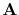, and the load vector,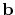, have to be determined. However, instead of computing them using directly (4.11) and (4.12), in practice they are computed by summing the contributions from the different elements [152,153,154] according to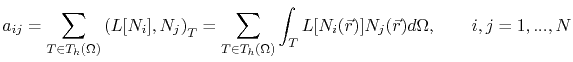(4.13)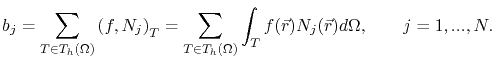(4.14)

Note that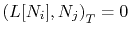unless both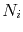and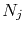belong to the same element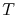. Thus, the calculations (4.13) and (4.14) can be limited to the nodes of the element, so that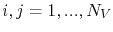, where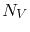is the number of vertices of the element. In this way, for each element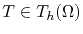, a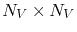matrix is obtained, which is called element stiffness or nucleus matrix. Thus, the general system matrix,, can be computed by first computing the nucleus matrices for eachand then summing the contributions from each element according to (4.13) . The right-hand side vector,, is computed in the same way. This process of constructing the general system matrix is called assembly . The main advantage of this assembly process is that it greatly simplifies the computation of the system matrix and right-hand side vector, since (4.11) and (4.12) can be easily calculated for each element of the domain discretization.Next: 4.1.3 Shape Function Up: 4.1 The Finite Element Previous: 4.1.1 Galerkin's Method

R. L. de Orio: Electromigration Modeling and Simulation